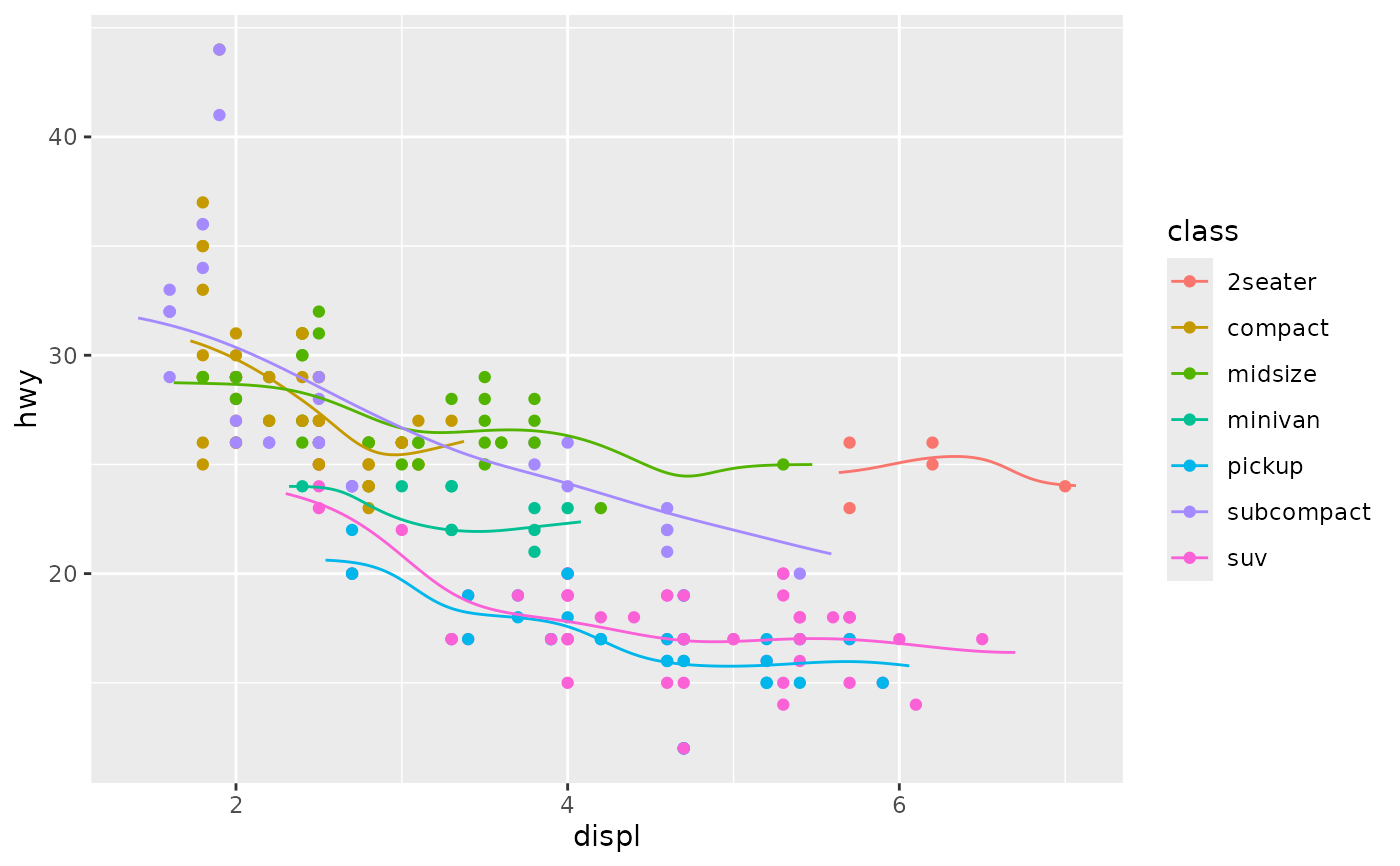A rolling kernel moves along one of the axes and assigns weights to datapoints depending on the distance to the kernel's location. It then calculates a weighted average on the y-values of the datapoints, creating a trendline. In contrast to (weighted) rolling averages, the interval between datapoints do not need to be constant.

## Usage

stat_rollingkernel(
mapping = NULL,
data = NULL,
geom = "line",
position = "identity",
...,
bw = "nrd",
kernel = "gaussian",
n = 256,
expand = 0.1,
na.rm = FALSE,
orientation = "x",
show.legend = NA,
inherit.aes = TRUE
)

## Arguments

mapping

Set of aesthetic mappings created by aes(). If specified and inherit.aes = TRUE (the default), it is combined with the default mapping at the top level of the plot. You must supply mapping if there is no plot mapping.

data

The data to be displayed in this layer. There are three options:

If NULL, the default, the data is inherited from the plot data as specified in the call to ggplot().

A data.frame, or other object, will override the plot data. All objects will be fortified to produce a data frame. See fortify() for which variables will be created.

A function will be called with a single argument, the plot data. The return value must be a data.frame, and will be used as the layer data. A function can be created from a formula (e.g. ~ head(.x, 10)).

geom

Use to override the default geom ("line").

position

Position adjustment, either as a string naming the adjustment (e.g. "jitter" to use position_jitter), or the result of a call to a position adjustment function. Use the latter if you need to change the settings of the adjustment.

...

Other arguments passed on to layer(). These are often aesthetics, used to set an aesthetic to a fixed value, like colour = "red" or size = 3. They may also be parameters to the paired geom/stat.

bw

A bandwidth, which can be one of the following:

• A numeric of length one indicating a measure of kernel width, in data units.

• A rel object of length one constructed for setting a bandwidth relative to the group data range. Can be constructed with the rel() function.

• A character of length one, naming one of the functions documented in bw.nrd().

kernel

One of the following:

• A function that takes a vector of distances as first argument, a numeric bandwidth as second argument and returns relative weights.

• A character of length one that can take one of the following values:

"gaussian" or "norm"

A kernel that follows a normal distribution with 0 mean and bandwidth as standard deviation.

"mean" or "unif"

A kernel that follows a uniform distribution with $$bandwidth * -0.5$$ and $$bandwidth * 0.5$$ as minimum and maximum. This is similar to a simple, unweighted moving average.

"cauchy"

A kernel that follows a Cauchy distribution with 0 as location and bandwidth as scale parameters. The Cauchy distribution has fatter tails than the normal distribution.

n

An integer of length one: how many points to return per group.

expand

A numeric of length one: how much to expand the range for which the rolling kernel is calculated beyond the most extreme datapoints.

na.rm

If FALSE, the default, missing values are removed with a warning. If TRUE, missing values are silently removed.

orientation

A character of length one, either "x" (default) or "y", setting the axis along which the rolling should occur.

show.legend

logical. Should this layer be included in the legends? NA, the default, includes if any aesthetics are mapped. FALSE never includes, and TRUE always includes. It can also be a named logical vector to finely select the aesthetics to display.

inherit.aes

If FALSE, overrides the default aesthetics, rather than combining with them. This is most useful for helper functions that define both data and aesthetics and shouldn't inherit behaviour from the default plot specification, e.g. borders().

## Value

A Layer ggproto object.

## Aesthetics

stat_rollingkernel() understands the following aesthetics (required aesthetics are in bold)

• x

• y

• group

## Computed variables

x

A sequence of ordered x positions.

y

The weighted value of the rolling kernel.

weight

The sum of weight strengths at a position.

scaled

The fraction of weight strengths at a position. This is the same as weight / sum(weight) by group.

## Examples

ggplot(mpg, aes(displ, hwy, colour = class)) +
geom_point() +
stat_rollingkernel()# The (scaled) weights can be used to emphasise data-dense areas
ggplot(mpg, aes(displ, hwy, colour = class)) +
geom_point() +
stat_rollingkernel(aes(alpha = after_stat(scaled)))